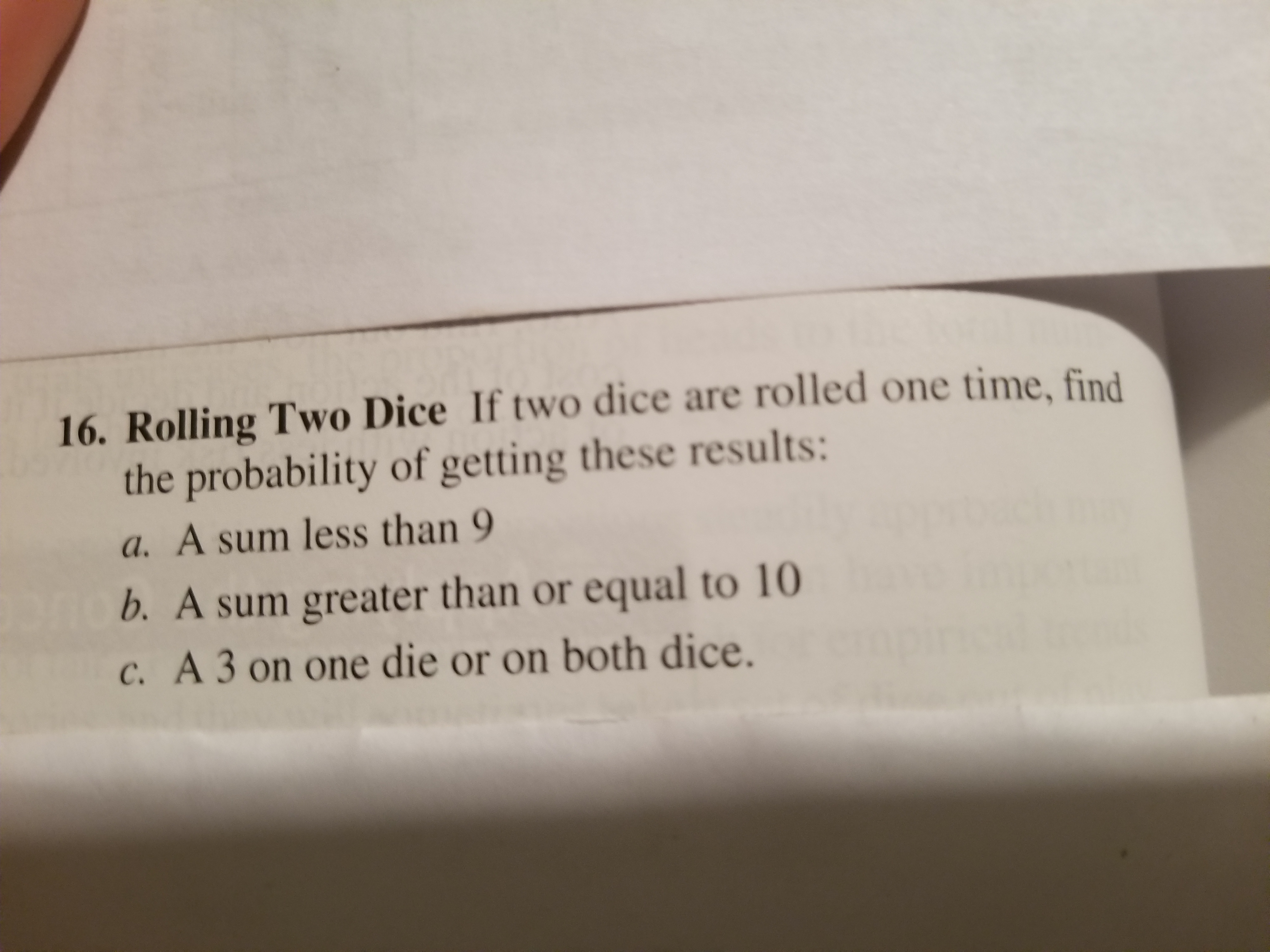# 16. Rolling Two Dice If two dice are rolled one time, findthe probability of getting these results:a. A sum less than 9b. A sum greater than or equal to 10c. A 3 on one die or on both dice.

Question
192 viewshelp_outlineImage Transcriptionclose16. Rolling Two Dice If two dice are rolled one time, find the probability of getting these results: a. A sum less than 9 b. A sum greater than or equal to 10 c. A 3 on one die or on both dice. fullscreen
check_circle

Step 1

Concept of probability:

Probability deals with the likelihood of occurrence of a given event. The probability value lies between 0 and 1. An event with probability 1 is considered as certain event and an event with probability 0 is considered as an impossible event. The probability of 0.5 infers of having equal odds of occurring and not occurring of an event.

The general formula to obtain probability of an event A is,

P(A) = (number of favorable elements for event A)/(Total number of elements in the sample space).

The basic properties of probability are given below:

Step 2

Find the sample space:

Sample space:

The set of all possible outcomes of a probability experiment is called sample space of the experiment.

Here, two fair dice are rolled.

The total number of outcomes in the sample space be n(S) = 62 = 36. That is, there will be 36 equally likely outcomes.

Outcomes will be occurred in ordered pairs. First number in each ordered pair represents the number on the first die and second number in each ordered pair represents the number on the second die. Each of the two numbers can take values 1 to 6.

The sum of the numbers on the dice will be 2, 3, 4, 5, 6, 7, 8, 9, 10 and 11.

The sample space for the experiment is given below:

Step 3

Find the probability that the sum of the numbers on the pair of rolled die is less than 9:

Here, the requirement is that, the sum of the numbers on the pair of rolled die should be less than 9.

Consider that an event A denotes that, the sum of the dice is less than 9.

The outcomes in favor of the event A:

Event A is the sum of the dice is less than 9. That is, the sum must be any of 2, 3, 4, 5, 6, 7 and 8.

The outcomes in the event A consist of all the possible outcomes for the sum of the dice to be 2, 3, 4, 5, 6, 7 and 8.

The outcomes in favo...

### Want to see the full answer?

See Solution

#### Want to see this answer and more?

Solutions are written by subject experts who are available 24/7. Questions are typically answered within 1 hour.*

See Solution
*Response times may vary by subject and question.
Tagged in

### Other